﻿ A New Numerical Method for Solving First Order Differential Equations

### A New Numerical Method for Solving First Order Differential Equations

Ayinde S. O., Ibijola E. A.

## A New Numerical Method for Solving First Order Differential Equations

Ayinde S. O.1,, Ibijola E. A.1

1Department of Mathematical Sciences, Faculty of Science, Ekiti State University, Ado Ekiti, Nigeria

### Abstract

In this paper, we present a new numerical method for solving first order differential equations. The new numerical integration scheme was obtained which is particularly suited to solve oscillatory and exponential problems. We verify the reliability of the new scheme and the results obtained show that the scheme is computationally reliable, and competes favourably with other existing ones.

• Ayinde S. O., Ibijola E. A.. A New Numerical Method for Solving First Order Differential Equations. American Journal of Applied Mathematics and Statistics. Vol. 3, No. 4, 2015, pp 156-160. http://pubs.sciepub.com/ajams/3/4/4
• O., Ayinde S., and Ibijola E. A.. "A New Numerical Method for Solving First Order Differential Equations." American Journal of Applied Mathematics and Statistics 3.4 (2015): 156-160.
• O., A. S. , & A., I. E. (2015). A New Numerical Method for Solving First Order Differential Equations. American Journal of Applied Mathematics and Statistics, 3(4), 156-160.
• O., Ayinde S., and Ibijola E. A.. "A New Numerical Method for Solving First Order Differential Equations." American Journal of Applied Mathematics and Statistics 3, no. 4 (2015): 156-160.

 Import into BibTeX Import into EndNote Import into RefMan Import into RefWorks

12
Prev Next

### 1. Introduction

We shall consider the initial value problem of the form(1)

Which in time past, many scholars have derived different polynomial integrating functions to produce schemes capable of solving ordinary differential equations. Therefore we shall developed numerical scheme to solve problem (1) in tune with those developed by Fatunla [1, 2], Ibijola , and Ogunrinde et.al .

### 2. Derivation of New Scheme

The mathematical formulation of physical phenomena in almost every sphere of human endeavor, be it engineering, control theory, biological, and economics often leads to one or a set of nth order differential equations in the form

Let us assume that the theoretical solution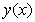to the initial value problem (1) can be locally represented in the intervalby the non-polynomial interpolating function;(2)

Where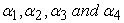are real undetermined coefficients and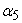is a constant. We shall assumeis a numerical estimate to the theoretical solutionand.

We define mesh points as follows: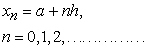(3)

Imposing the following constraints on the interpolating function (2) in order to get the undetermined coefficients.

2.1. The Interpolating Function.

a. The interpolating function must coincide with the theoretical solution atHence we required that(4)(5)

b. Secondly, the derivatives of the interpolating function are required to coincide with the differential equation as well as its first, second, and third derivatives with respect to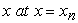.We denote the ith total derivatives ofwith respect towith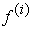such that(6)(7)(8)
2.2. The Derivatives of the Interpolant(9)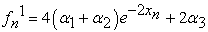(10)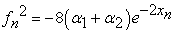(11)

Solving for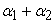from equation (11), we have(12)

Substituting (12) into (10), we have(13)

Substituting (12) and (13) into (9), we have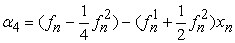(14)

Since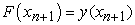andImplies that(15)Then we shall have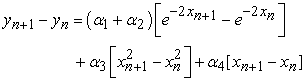(16)

Recall that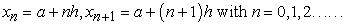(17)

Substitute (12), (13) and (14) into (16), we have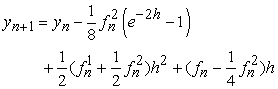(18)

Hence (18) is the new schemes for solution of the first order differential equation.

### 3. The Implementation of the Scheme

Example 1

#### Table 3.1Download asVeiw tableTables index

Hence the graph of Table 3.1Download asVeiw figureFigures index
Figure
Example 2.

#### Table 3.2Download asVeiw tableTables index

Hence the graph of Table 3.2Download asVeiw figureFigures index
Figure
Example 3

#### Table 3.3Download asVeiw tableTables index

Hence the graph of Table 3.3Download asVeiw figureFigures index
Figure
Example 4

#### Table 3.4Download asVeiw tableTables index

Hence the graph of Table 3.4Download asVeiw figureFigures index
Figure

### 4. Summary and Conclusion

The following can be deduced from this numerical experiment as summary and conclusion

(i) The results obtained from the implementation of the scheme revealed that the approximation/numerical solution and the exact/theoretical solution obtained leads to error that is miniature in size.

(ii) The smaller the step-size “h” the more accurate is the numerical methods.

(iii) It can be clearly seen from the results that the scheme can be used to solve any standard initial value problems as the scheme converges easily.

(iv) Since the error is not blown off, it showed the reasonability of the stability of the scheme.

### References

  Fatunla, S. O., (1976). A New Algorithm for the Numerical Solution of ODEs. Computers and Mathematics with Applications. USA. 2, 247-253.In article View Article  Fatunla, S. O., (1988). Numerical Methods for initial Value Problems in Ordinary Differential Equations, Academic Press, San Diego, U. S.A.In article  Ibijola, E. A., (1997). New Schemes for Numerical Integration of Special Initial Value Problems in Ordinary Differential Equations. Ph.d Thesis, University of Benin, Nigeria.In article  Ogunrinde, R.B., Fadugba, S.E., and Okunlola J. T. (2012). On some Numerical methods for solving initial value problem in ODEs. IOSR Journal of Mathematics (IOSRJM). 1(3), 25-31.In article View Article  Kama, P. and Ibijola, E. A. (2000). On a New One – Step Method for Numerical Integration of Ordinary Differential Equtions. International Journal of Computer Mathematics, Vol.78, No. 3,4.In article  Lambert, J. D. (1991). Numerical methods for Ordinary Differential Systems: the initial value problem. John Wiley & Sons, Inc., New York.In article  Shepley L. Ross (1984). Differential Equations. Third Edition. John Wiley & Sons, Inc., New York.In article